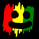4481 뷰
Uses 4 Ma cross...
Double Hull Moving Averages
Volume Weighted Moving Average
Weighted Moving Average

<<<<< by SeaSide420 >>>>>>
```//@version=2
//                                                    DoubleHullMA & VWMA & WMA combination  <<<<< by SeaSide420 >>>>>>
strategy("Multi-MA Strategy", overlay=true)
keh=input(title="Double HullMA",type=integer,defval=3, minval=1)
teh=input(title="Volume-Weighted MA",type=integer,defval=3, minval=1)
yeh=input(title="Base Weighted MA",type=integer,defval=75, minval=1)
rvwma=vwma(close,round(teh)),yma=wma(close,round(yeh)),n2ma=2*wma(close,round(keh/2)),nma=wma(close,keh)
diff=n2ma-nma,sqn=round(sqrt(keh)),n2ma1=2*wma(close,round(keh/2)),nma1=wma(close,keh)
diff1=n2ma1-nma1,sqn1=round(sqrt(keh)),n1=wma(diff,sqn),n2=wma(diff1,sqn)
b=n1>n2?lime:red,c=n1>n2?green:red,d=n1>rvwma?green:red,e=rvwma>yma?green:red
a1=plot(n1,color=c),a2=plot(n2,color=c),a3=plot(yma,color=e),vwma=plot(rvwma,color=d, linewidth = 2)
plot(cross(n1, n2) ? n1 : na, style = cross, color=b, linewidth = 3)
closelong = n1<n2 and n1>yma and n1<rvwma
if (closelong)
strategy.close("Long")
closeshort = n1>n2 and n1<yma and n1>rvwma
if (closeshort)
strategy.close("Short")
longCondition = n1>n2 and strategy.opentrades<1 and n1<yma and n1>rvwma
if (longCondition)
strategy.entry("Long",strategy.long)
shortCondition = n1<n2 and strategy.opentrades<1 and n1>yma and n1<rvwma
if (shortCondition)
strategy.entry("Short",strategy.short)```

## 코멘트vaicru
I made it on 4H (NZDUSD), unknown results of other pairs/timeframes, try it, just play with the settings.
New strategy on its way, same as this, but with Stoch,MacD & Ichimoku added to its open/close conditions.
응답SeaSide420
seems better once the strategy setting "recalculate after order filled" is ticked
Order size set to "% of equity"
Pyramiding will not work with this strategy because of the script line "strategy.opentrades<1" (only opens a new position if opentrades is less than 1 (zero/none)
응답SeaSide420
seems the other name for "recalculate after order filled" is "i know the future for one candle ahead of time" - almost all of my strategies look fantasticly profitable when the setting is ticked. so sad i don't know the future...
응답
프로화일 프로화일설정 계정 및 빌링 프렌드 리퍼하기 나의 서포트 티켓 헬프 센터 공개아이디어 팔로어 팔로잉 비밀메시지 채팅 로그아웃